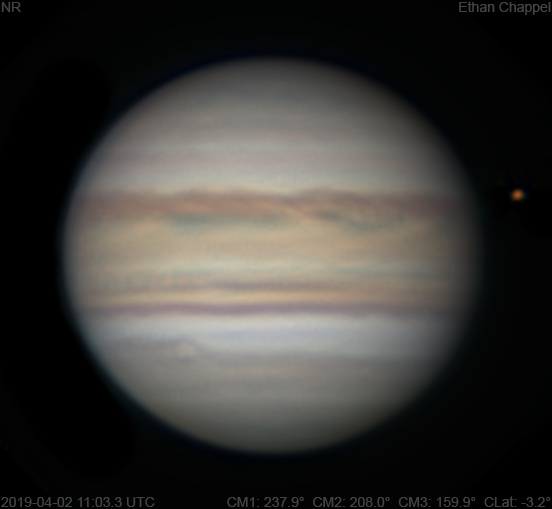# Jupiter 2019-04-02 11:03 UTC

CM1: 237.90°

CM2: 208.00°

CM3: 159.90°

CLat: -3.20°

Description

Another morning, another imaging session near my university's parking lot, and a surprisingly cold one at that. In fact, the forecasts I checked the evening before were ~10°C warmer than the actual temperature! This time, I had my 3x barlow and the right plugs to avoid using the power strip with my Power Tank.

Notes:

• There is a complex of small rifts on the North Equatorial Belt, starting at the central meridian and stretching eastward.
• Oval BA and the STB Ghost are rising.
• The Equatorial Zone is orange.
• Oval BA has lost its red color after having it for over a decade.

Equipment

ZWO ASI174MM

Celestron X-Cel 3x

Celestron EdgeHD 8

Logs
```FireCapture v2.6  Settings
------------------------------------
Observer=Ethan Chappel
Camera=ZWO ASI174MM
Filter=R
Profile=Jupiter
Diameter=39.98"
Magnitude=-2.26
CMI=235.3° CMII=205.5° CMIII=157.4°  (during mid of capture)
FocalLength=7700mm (F/27)
Resolution=0.16"
Filename=2019-04-02-1100_0-EC-R-Jup.ser
Date=2019_04_02
Start=10_59_05.676
Mid=11_00_05.683
End=11_01_05.690
Start(UT)=10_59_05.676
Mid(UT)=11_00_05.683
End(UT)=11_01_05.690
Duration=120.014s
Date_format=yyyy_MM_dd
Time_format=HH_mm_ss
LT=UT -6h
Frames captured=3994
File type=SER
Binning=no
Bit depth=8bit
Debayer=no
ROI=420x428
ROI(Offset)=0x0
FPS (avg.)=33
Shutter=30.00ms
Gain=400 (100%)
AutoExposure=off
AutoHisto=75 (off)
SoftwareGain=10 (off)
FPS=100 (off)
Brightness=1 (off)
AutoGain=off
Gamma=50 (off)
USBTraffic=80 (off)
HighSpeed=off
Histogramm(min)=0
Histogramm(max)=113
Histogramm=44%
Noise(avg.deviation)=2.57
AutoAlign=false
PreFilter=none
Limit=120 Seconds
Sensor temperature=20.8°C
FireCapture v2.6  Settings
------------------------------------
Observer=Ethan Chappel
Camera=ZWO ASI174MM
Filter=B
Profile=Jupiter
Diameter=39.98"
Magnitude=-2.26
CMI=239.5° CMII=209.7° CMIII=161.6°  (during mid of capture)
FocalLength=7900mm (F/28)
Resolution=0.15"
Filename=2019-04-02-1106_9-EC-B-Jup.ser
Date=2019_04_02
Start=11_05_56.677
Mid=11_06_56.693
End=11_07_56.710
Start(UT)=11_05_56.677
Mid(UT)=11_06_56.693
End(UT)=11_07_56.710
Duration=120.033s
Date_format=yyyy_MM_dd
Time_format=HH_mm_ss
LT=UT -6h
Frames captured=2997
File type=SER
Binning=no
Bit depth=8bit
Debayer=no
ROI=420x428
ROI(Offset)=0x0
FPS (avg.)=24
Shutter=40.00ms
Gain=340 (85%)
AutoExposure=off
AutoHisto=75 (off)
SoftwareGain=10 (off)
FPS=100 (off)
Brightness=1 (off)
AutoGain=off
Gamma=50 (off)
USBTraffic=80 (off)
HighSpeed=off
Histogramm(min)=0
Histogramm(max)=104
Histogramm=40%
Noise(avg.deviation)=1.30
AutoAlign=false
PreFilter=none
Limit=120 Seconds
Sensor temperature=20.2°C
FireCapture v2.6  Settings
------------------------------------
Observer=Ethan Chappel
Camera=ZWO ASI174MM
Filter=G
Profile=Jupiter
Diameter=39.98"
Magnitude=-2.26
CMI=237.2° CMII=207.3° CMIII=159.2°  (during mid of capture)
FocalLength=7700mm (F/27)
Resolution=0.16"
Filename=2019-04-02-1103_0-EC-G-Jup.ser
Date=2019_04_02
Start=11_02_04.600
Mid=11_03_04.615
End=11_04_04.630
Start(UT)=11_02_04.600
Mid(UT)=11_03_04.615
End(UT)=11_04_04.630
Duration=120.030s
Date_format=yyyy_MM_dd
Time_format=HH_mm_ss
LT=UT -6h
Frames captured=4793
File type=SER
Binning=no
Bit depth=8bit
Debayer=no
ROI=420x428
ROI(Offset)=0x0
FPS (avg.)=39
Shutter=25.00ms
Gain=360 (90%)
AutoExposure=off
AutoHisto=75 (off)
SoftwareGain=10 (off)
FPS=100 (off)
Brightness=1 (off)
AutoGain=off
Gamma=50 (off)
USBTraffic=80 (off)
HighSpeed=off
Histogramm(min)=0
Histogramm(max)=126
Histogramm=49%
Noise(avg.deviation)=1.64
AutoAlign=false
PreFilter=none
Limit=120 Seconds
Sensor temperature=20.5°C
```